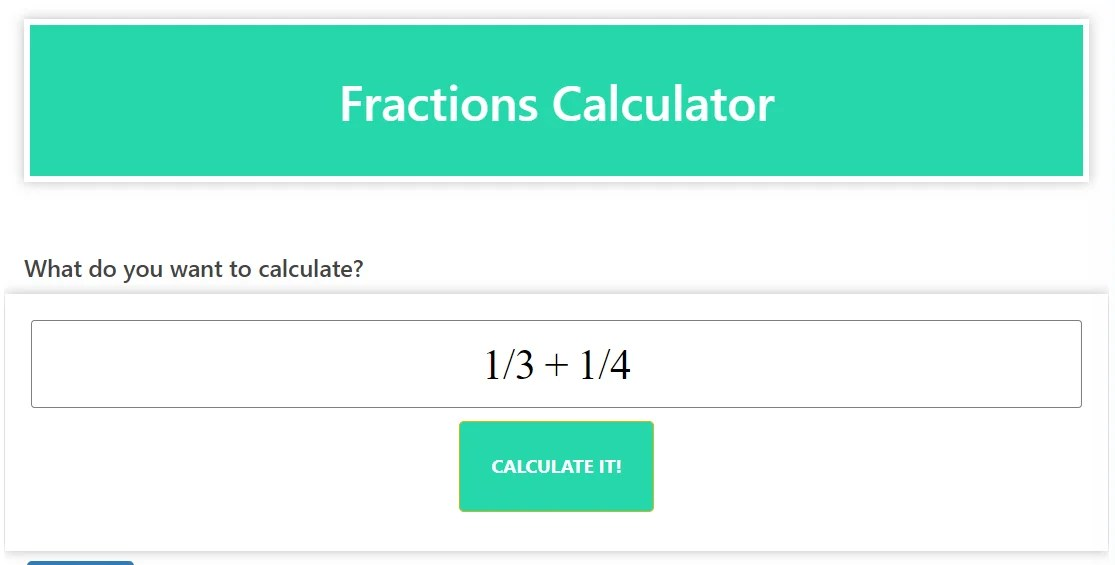# Fraction Calculator (Maths Solver): Unlocking the Power of Fraction Computations

The fractions Calculator, also known as Maths Solver, is a versatile tool designed to simplify the process of working with fractions. Whether you are a student learning fractions, a professional dealing with fractional values, or an enthusiast in need of accurate and efficient solutions, our fraction calculator is here to help.

### INTUITIVE FRACTION COMPUTATIONS:

Working with fractions can be complicated and time-consuming, but our fractions calculator streamlines the process by automating the computations. Simply input the fractions and the desired operation (addition, subtraction, multiplication, or division), and our calculator will provide the result in the simplest form.

### Versatile Fraction Operations:

The fractions calculator can handle a wide range of fraction operations, accommodating both proper and improper fractions. It allows you to do addition, subtraction, multiplication, and division with ease. The calculator considers the numerator, denominator, and any mixed number components to give accurate and reliable results.

### Step-by-Step Solutions:

Understanding the step-by-step process of fraction computations is important to master the concepts. With our fraction calculator, you get not only the final result but also insight into the detailed solution. Each calculation is accompanied by a comprehensive explanation, so you can follow along and deepen your understanding of fraction operations.

### Efficiency and accuracy:

Time is valuable, and our fractions calculator ensures both efficiency and accuracy. Automating different calculations saves you valuable time and reduces the risk of errors. Rest assured that the calculator provides accurate and reliable results, giving you confidence in the accuracy of your calculations.

### UNLOCK THE POWER OF THE FRACTION CALCULATOR:

Whether you are a student learning fractions, a professional working with fractional values, or an enthusiast exploring the world of math, our fractions calculator is an invaluable resource. Simplify your fraction calculations and unlock new possibilities in mathematical problem-solving.

Experience the benefits of a Fractions Calculator: Change your approach to fraction operations with our advanced Fractions Calculator. Say goodbye to manual calculations and embrace the efficiency and accuracy of our automated tools. Empower yourself with a calculator that simplifies complex fractions calculations. Explore the possibilities with our fraction calculator and boost your math skills today.